Mobile QR Code1. (School of Electrical and Computer Engineering, University of Seoul, Seoul, Korea)

Opamp-sharing technique, capacitor-sharing technique, comparator-sharing technique, auto-zeroing, pipelined ADC

## I. INTRODUCTION

As CMOS process technology has advanced considerably over decades and system-on-chip has been a huge demand, design trend of integrated circuits requires lower power consumption and smaller die area simultaneously. On the other hand, higher specifications of operating speeds and resolutions should become more significant because wireless communication systems and high definition image processing require a higher level of performance. Various types of ADCs are widely available in order to achieve the aforementioned requirements .

In a pipelined ADC, an operational amplifier (opamp) must be the most critical component for achieving the required performance. This makes an operational amplifier the most demanding block in terms of power dissipation and hardware area resource. Many techniques that reduce power consumption and die area have been developed over the years as technology has advanced. Among these techniques, switched-opamp technique, SHA-less configuration, opamp-sharing method, and capacitor-sharing techniques are well known.

The switched-opamp technique is widely used to save power consumption for switched-capacitor applications [2-4]. The main purpose of this technique is to turn off the operational amplifier during the input-sampling phase when the amplifier is not in use. This technique, however, requires a fast settling time during the amplifying phase. Although this technique is suitable for saving energy, the number of amplifiers is not changed.

In constructing a pipelined ADC, most power and area budgets are limited by the sample-and-hold amplifier. In order to alleviate power and die area requirements, various SHA-less structures have been developed in [5-9]. Even though power consumption is significantly reduced with the absence of a SHA circuit, this approach suffers from an aperture error due to sampling-time mismatch between the input sampling networks of the Multiplying DAC (MDAC) and flash ADC (FADC) in the first stage, which might lead to a missing code problem. In order to minimize mismatch, an elaborate design is required by considering a signal path in layout.

Further reduction in power consumption and die area can be achieved by sharing operational amplifiers between adjacent MDAC stages. Since every stage consists of an MDAC, sharing operational amplifiers should be one of the most efficient ways for pipelined ADCs. This technique, however, has a couple of drawbacks. First, settling time is degraded by RC time delay that results from additional switches for amplifier sharing. Second, memory effect caused by the input parasitic capacitance affects the output at the current operating phase. In order to alleviate the memory effect, adding output sampling capacitors [10,11], putting discharge phase [12,13], using dual pairs of input stages [14-16], and sharing the output stage of amplifier were proposed .

In this paper, novel techniques that reduce both power consumption and die area are proposed. Combination ofSHA and MDAC at the first stage and the comparator sharing techniqueare presented. The ADC architecture using the proposed circuit-sharing techniques is introduced in Section II. The detailed circuit implementation is described in Section III. Measurement results of the prototype ADC are shown in Section IV. The conclusion is given in Section V.

## II. PROPOSED ADC ARCHITECTURE USING CIRCUIT-SHARING TECHNIQUES

The block diagram of the proposed 11-bit pipelined ADC architecture is shown in Fig. 1. The ADC consists of four pipeline stages, digital correction logic (DCL), clock generator, and bias/reference generator circuit. In the first stage, SHA and 2.5-bit MDAC are combined with the proposed amplifier and capacitor sharing technique. Consecutively, Two 3.5-bit MDAC stages are merged with the conventional opamp-sharing technique. Moreover, first two 2.5-bit FADCs and next two 3.5-bit FADCs are combined respectively by sharing comparators between the adjacent stages.

### 1. Proposed Technique of SHA & $1^{st}$ MDAC

Since a sample-and-hold amplifier is located at the front-end of a pipelined ADC, it occupies a large portion of power dissipation and die area. The conventional sharing technique for a SHA-less structure shown in Fig. 2(a) has several drawbacks. First, the offset voltage of an operational amplifier cannot be removed. Second, the output voltage during a hold phase is determined by the ratio between the sampling capacitor and feedback capacitor. Third, the corresponding feedback factor deteriorates the operational speed of the closed-loop amplifier. Finally, a reset phase for the input stage might be required to avoid the memory effect.

Fig. 1. Block diagram of the proposed 11-bit pipelined ADC.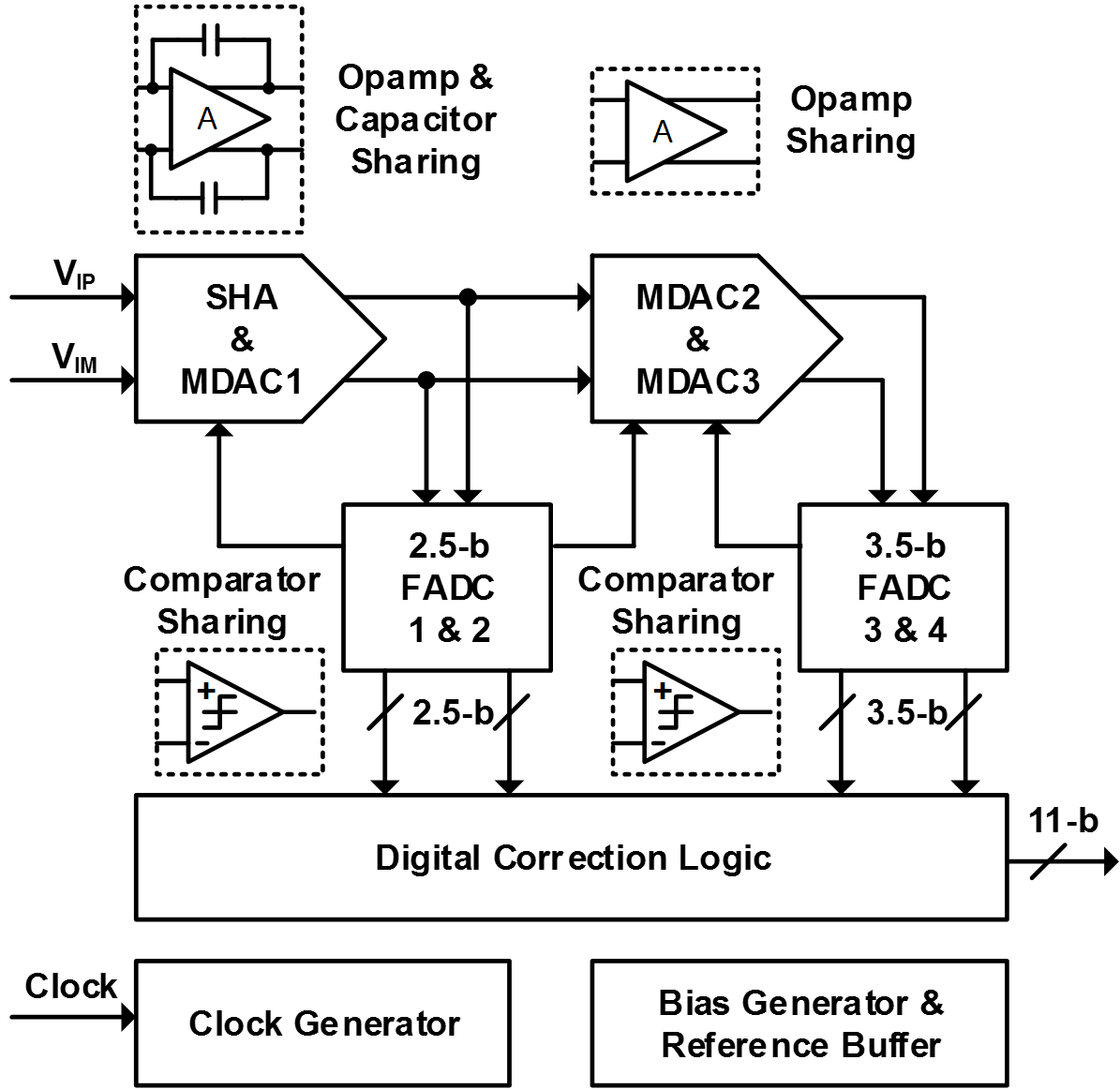Fig. 2. Opamp and capacitor sharing techniques between SHA and MDAC (a) Conventional approach, (b) Proposed technique.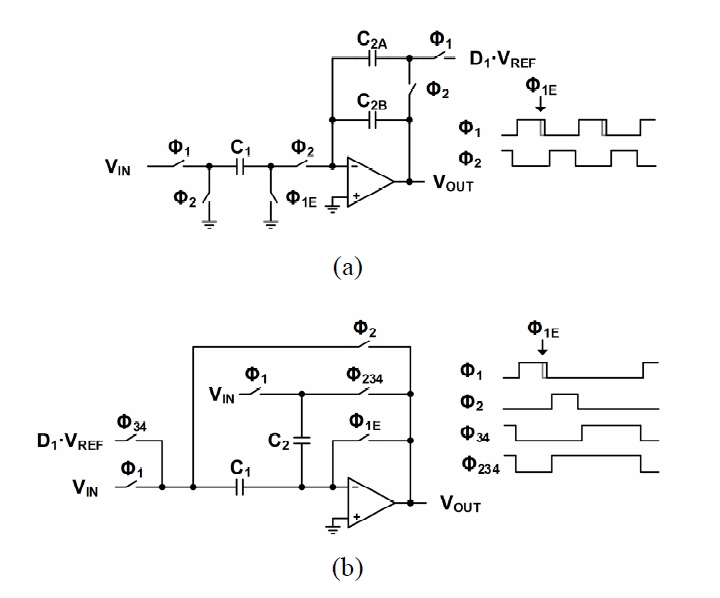In order to remedy these drawbacks, the new amplifier and capacitor sharing technique is proposed as shown in Fig. 2(b). The flip-around SHA and MDAC circuit is implemented with sharing amplifier and capacitors. The circuit employs three operation phases for sampling ($Φ_1$), hold ($Φ_2$) and amplification ($Φ_{34}$). The offset voltage of anamplifier can be removed and output voltage during the hold phase is independent of the ratio of capacitors owing to the characteristics of a flip-around SHA. Unlike the conventional sharing technique that adopts charge-redistribution amplifier type, there is no gain error due to capacitance mismatch. In addition, maximum feedback factor of 1 can be achieved if input parasitic capacitance is ignored, which can improve the operation speed.

Fig. 3. Operations of the proposed technique with input parasitic capacitance (a) Sampling phase ($Φ_1$), (b) Hold phase ($Φ_2$), (c) Amplification phase ($Φ_{34}$).While the operational amplifier is able to be reset during the sampling phase, the circuit might suffer from the memory effect because there is no reset after the hold phase. To examine this error, the simplified operations are illustrated in Fig. 3, where $D_1$ is the input of MDAC that results from the FADC in the same stage. The output voltage at the hold phase $Φ_2$, $V_{HOLD}$ is derived as,

##### (1)
$V_{HOLD}\approx V_{IN}\cdot \left[1- \left(1+\frac{C_{P}}{C_{1}+C_{2}}\right)\cdot \frac{1}{A}\right]$

where $C_P$ and A are the input parasitic capacitor and finite gain of the operational amplifier, respectively. The output voltage during the amplification phase $Φ_{34}$, $V_{RES}$ can be also derived as,

##### (2)
$V_{RES}\approx \frac{C_{1}+C_{2}}{C_{2}}\cdot \left(1- \frac{C_{1}}{C_{1}+C_{2}}\cdot \frac{C_{1}+C_{2}+C_{P}}{C_{2}}\cdot \frac{1}{A}\right)\cdot V_{HOLD}\\ - \frac{C_{1}}{C_{2}}\cdot \left(1- \frac{C_{1}+C_{2}+C_{P}}{C_{2}}\cdot \frac{1}{A}\right)\cdot D_{1}\cdot V_{REF}$

Fig. 4. Circuit schematic of the proposed 2.5-bit comparator-sharing FADC.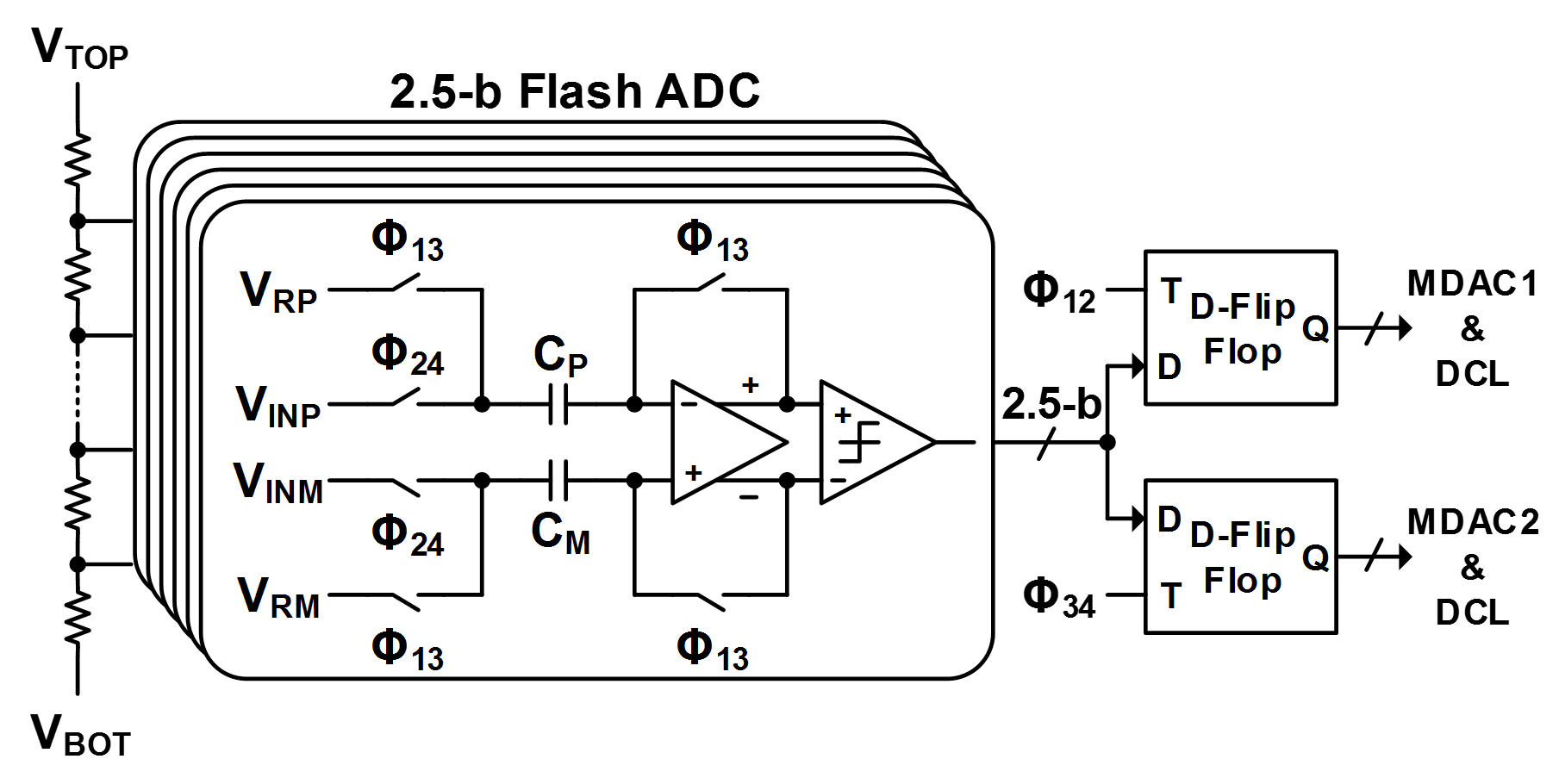In (2), it is shown that the memory effect caused by the input parasitic capacitance translates into a gain-error term. On the other hand, the residue voltage, $V_{RES}$ of a conventional MDAC is calculated as

##### (3)
$V_{RES}\approx \frac{C_{1}+C_{2}}{C_{2}}\cdot \left(1- \frac{C_{1}+C_{2}+C_{P}}{C_{2}}\cdot \frac{1}{A}\right)\cdot V_{HOLD}\\ - \frac{C_{1}}{C_{2}}\cdot \left(1- \frac{C_{1}+C_{2}+C_{P}}{C_{2}}\cdot \frac{1}{A}\right)\cdot D_{1}\cdot V_{REF}$

By comparing (2)and (3), it can be shown that the gain error term related to the MDAC input voltage $V_{HOLD}$ is reduced by $C_1$ / ($C_1$ + $C_2$) when the proposed approach is applied. In this work, the capacitors $C_1$ and $C_2$ have a 3:1 ratio. Thus, a 25% decrease in gain-error can be achieved.

### 2. Proposed Comparator-sharing Flash ADC

In a conventional pipelined ADC, a FADC is used to convert the output signal of the previous stage to digital code. The converted signal is transferred to the MDAC for residue signal processing and DCL for error correction. The number of FADC can be reduced by sharing the building components to save power consumption as well as the hardware area. This sharing can be possible by operation with 2 times faster clock. In the recent deep sub-micron technology, operating the comparators with doubled clock frequency can be acceptable without major penalty of power consumption increase under the condition that the operational speed is limited by the operational amplifier, not the comparator. The proposed sharing technique is shown in Fig. 4.

During both $Φ_1$ and $Φ_3$ phases as shown in $Φ_{13}$, the FADC samples reference voltages from a resistor array and all pre-amplifiers are reset. During both $Φ_2$ and $Φ_4$ phases as shown in $Φ_{24}$, the difference between the reference voltage and output voltage is amplified by the pre-amplifier and transferred to the latched comparator that generates the digital code. The output D-flip flops are used to retain the digital code and synchronize the clock cycles for DAC operation in the corresponding MDAC. In this pipelined ADC, a 2.5-bit comparator-sharing FADC is used for the first and second stages, and a 3.5-bit comparator-sharing FADC for the third and last stages. Timing diagram of the proposed pipelined ADC that incorporate the scheme of FADCs is shown in Fig. 5.

## III. CIRCUIT IMPLEMENTATION

### 1. Bandwidth Requirement of Operational Amplifier

Since the combined SHA and MDAC have different feedback configurations in different operating phases, the required bandwidth of the operational amplifier during each phase must be carefully investigated. As shown in Fig. 6, the combined SHA and MDAC have different load and feedback conditions during hold and amplification phases. During the hold phase in Fig. 6(a), the feedback factor of the closed loop becomes 1. Sampling capacitors are considered as load capacitors if input capacitors of the FADC are assumed to be negligible. Since the feedback factor is 1, the closed-loop bandwidth is the same as the unity-gain frequency of the operational amplifier, which is $g_{m1}/8C_U$, where $g_{m1}$ is the equivalent transconductance parameter of the amplifier.

Fig. 6. Bandwidth of the operational amplifier for the combined SAH and 1st MDAC stage during (a) hold phase, (b) amplification phase without capacitor scaling, (c) amplification phase with capacitor scaling.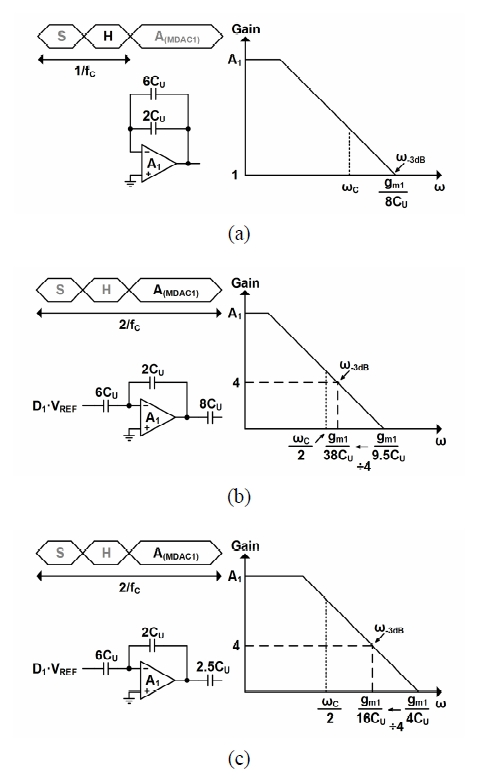On the other hand, during the amplification phase in Fig. 6(b), the feedback factor $\beta$ is reduced to 1/4 and the effective load is changed to $8C_U + 2C_U (1-\beta) = 9.5C_U$. In this case, the unity-gain frequency of the operational amplifier and closed-loop bandwidth are $g_{m1}/9.5C_U$ and $g_{m1}/38C_U$, respectively. Compared to the hold phase, the closed-loop amplifier operates at 2.3 times slower speed than the amplifier in the hold phase. This implies that the performance of the amplifier should be further optimized for the amplification phase. In order to avoid significantly increasing power consumption, capacitor scaling is suggested to reduce the power consumption . In order to maintain the same ratio of clock frequency to the closed-loop bandwidth, the effective load must be reduced to 4$C_U$. In other words, the sampling capacitor from the next stage must be scaled down from 8$C_U$ to 2.5$C_U$. The bandwidth requirement under the scaled-load condition is illustrated in Fig. 6(c).

Table 1. Sampling capacitor sizes of the proposed ADC

 Block Stage Sampling Capacitor Unit Capacitor Combined SHA&MDAC 1 1.52 pF 1.52 pF/8=190fF Opamp-Sharing MDAC 2 480 fF 480 fF / 8 = 60 fF 3 960 fF 960 fF / 16 = 60 fF 2.5-bit FADC 1 & 2 180 fF 180 fF/ 6 = 30 fF 3.5-bit FADC 3 &4 420 fF 420 fF / 14 = 30 fF Total Input-Reference Noise 6.6 $nV^2$

### 2. Consideration on Noise and Capacitor Scaling

Fundamental performance of an ADC is limited by various noise factors. While the quantization noise is determined by the resolution of ADC, the thermal noise is defined by kT/C noise, which means that the sampling capacitor must be large enough to keep the thermal noise less than the quantization one. However, this degrades the operating speed due to enlarged capacitance values. It is necessary to estimate the amount of noise in terms of the sampling capacitor sizes by setting a proper noise budget.

In our design, quantization noise power is calculated as 19.89 $nV^2$ where the full signal range is set to be 1 V. By setting the thermal noise budget to be 1/6 LSB (or equivalently 6.63 $nV^2$), signal-to-noise ratio (SNR) is obtained as 66.73 dB that is 1.25 dB less than an ideal SNR. From the given noise budget, the sampling capacitor size can be derived. With sharing technique of the operational amplifier and capacitors, the sampling phase of the first MDAC does not exist and corresponding thermal noise of the sampling circuit cannot be included for noise calculation. With the above-mentioned capacitor scaling for maintaining the bandwidth during different operating phases, the input-referred equivalent noise power can be derived as,

##### (4)
$\overline{V_{n.IN}^{2}}=2\cdot \left(\frac{kT}{C}+\frac{kT}{4^{2}\cdot 0.3125C}+\frac{kT}{4^{2}\cdot 4^{2}\cdot 0.625C}\right)\\ =2.41\frac{kT}{C}$

where factor of 2 is used for differential operations. Since the conventional four-stage pipelined ADC consisting of one SHA and three MDACs, this noise power is obtained as 4.13 kT/C, a minimum allowable capacitor size can be reduced in this proposed design, which is set to be 1.5 pF. The sampling capacitor sizes are shown in Table 1.

Fig. 7. Circuit schematic of the rail-to-rail fully differential folded-cascode amplifier with a gain boosting technique (a) Main amplifier, (b) Auxiliary amplifier AP for each PMOS cascode branch, (c) Auxiliary amplifier AN for each NMOS cascode branch.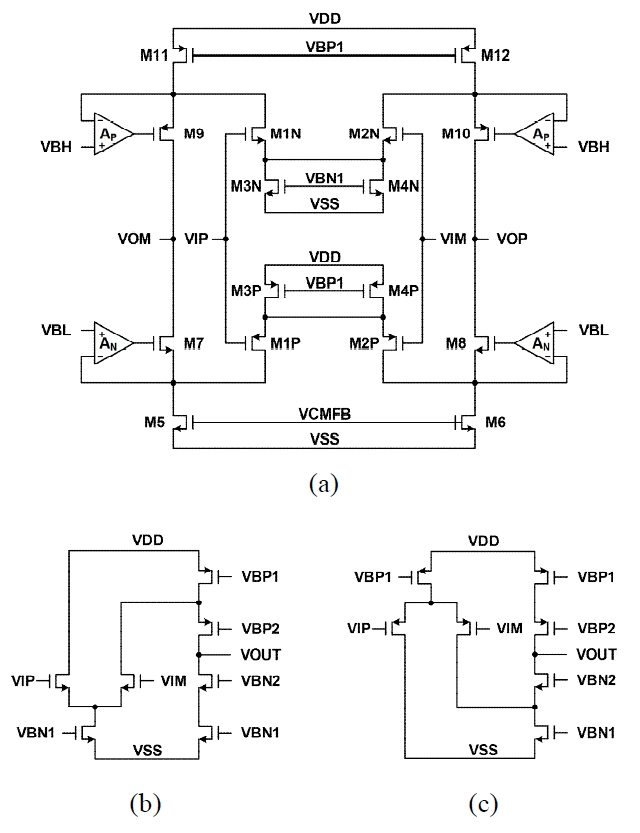### 3. Operational Amplifier

In low-voltage pipelined ADCs with the advanced processing technology, folded-cascode operational amplifiers suffer from reduced gain value due to shorter channel length of transistors and reduced voltage margin through drain-source terminals. Multi-stage configuration with various frequency compensation techniques can be usually adopted to enhance the amplifier voltage gain [19,20]. In spite of high DC gain, employing a multi-stage configuration is not adequate in high-speed applications because the closed-loop bandwidth should be limited for stability that might be degraded due to many poles. Even though various Miller compensation skills can be applied to push the second pole near or above the unity-gain frequency, settling behavior is still restricted by the compensation and load capacitor, and power consumption at the second and later stages are significantly increased for better phase margin.

In order to implement the operational amplifier that can satisfy both the above-mentioned bandwidth requirements and high DC gain for 11-bit resolution, a rail-to-rail fully differential folded-cascode amplifier with gain boosting scheme is used for the first and second stages. Using the modern advanced technology, gain-boosting technique is suitable with auxiliary amplifiers around each cascode branches for better stability and moderate power dissipation. The simulated DC gain of the amplifier is 92 dB. The circuit schematic of the main operational amplifier is shown in Fig. 7(a) and gain-boosting amplifiers for each PMOS and NMOS branch are shown in Fig. 7(b) and (c), respectively. The switched-capacitor common-mode feedback circuit is omitted for simplicity.

Fig. 10. Measured dynamic performance of the proposed ADC (a) FFT spectrum (8192 points), (b) SNDR and SFDR versus sampling and input frequencies.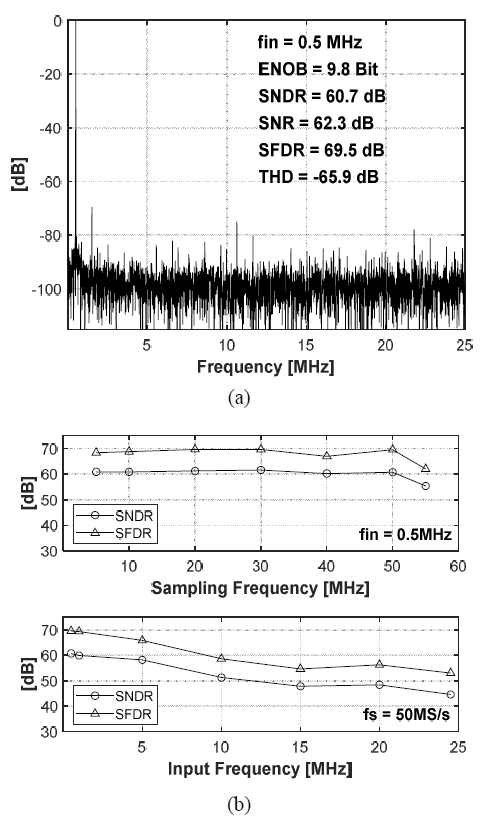## IV. MEASUREMENT RESULTS

The proposed 11-bit 50-MS/s prototype ADC was implemented in a 65-nm CMOS process. The die photo is shown in Fig. 8. The ADC occupies an active area of 0.62 $mm^2$. Measured static performance results are shown in Fig. 9. The differential nonlinearity (DNL) and integral nonlinearity (INL) are less than 0.6 and 1.1 LSB, respectively. Fig. 10(a) shows the measured FFT spectrum at conversion rate of 50 MS/s. The measured results show signal-to-noise and distortion ratio (SNDR) of 60.7 dB and spurious-free dynamic range (SFDR) of 69.5 dB. The effective number of bits is 9.8 for 0.5-MHz input. Dynamic performance results with respect to sampling and input frequencies are shown in Fig. 10(b).

Table 2. Performance summary and comparison

 This Work      Process [nm] 65 180 25 180 180 180 Resolution [bits] 11 10 11 10 10 12 Conversion Rate [MS/s] 50 40 20 50 50 50 Supply Voltage [V] 1.2 1.8 1.8 1.8 1.8 1.8 Input voltage Range [$V_{PP}$] 1 2 N/A 1.6 1 1.5 DNL / INL [LSB] 0.6 / 1.1 0.32 / 0.62 0.86 / 1.68 0.39 / 0.81 0.2 / 0.4 0.53 / 2.09 SNDR [dB] 60.7 59.53 61.8 58.4 56.9 60.6 SFDR [dB] 69.5 71.67 78.9 72.8 N/A 69.4 Power Consumption [mW] 10.8*/ 14.1** 14 2.24 12 18 21.6 Active Area [$mm^2$] 0.62 0.64 0.052 0.86 1.43 0.93 FoM [fJ/conv-step] 242*/ 316** 452 111 353 771 491

* without reference buffer

** with reference buffer

The proposed pipelined ADC achieves a figure of merit (FOM) value of 242 fJ/conversion-step. Measured performance results and comparison results with other significant prior works that employ opamp-sharing techniques with similar resolution and conversion rate are shown in Table 2.

## V. CONCLUSION

A novel operational amplifier and capacitor sharing technique is proposed for SHA and MDAC for the first stage of an 11-bit 50-MS/s pipelined ADC. This circuit can cancel the offset voltage of an operational amplifier and the gain error can be reduced with no memory effect. Comparator-sharing FADCs not only reduce the die area, but also improve linearity performance by sharing resistor ladders. The ADC is fabricated in a 65-nm CMOS technology. Measured performance of the ADC shows ENOB of 9.8 and power dissipation is 10.8 mW for a single supply voltage of 1.2 V.

### ACKNOWLEDGMENTS

This research was supported by the MSIT(Ministry of Science and ICT), Korea, under the ITRC(Information Technology Research Center) support program(IITP-2019-2018-0-01421) supervised by the IITP(Institute of Information & communications Technology Planning & Evaluation). The chip fabrication and EDA tool were supported by KAIST IC Design Education Center (IDEC), Korea.

### REFERENCES

1
Buchwald A., Apr 2016, High-speed time interleaved ADCs, IEEE Commun. Mag., Vol. 54, No. 4, pp. 71-772
Waltari M., Halonen K.A.I., Jan 2001, 1-V 9-bit pipelined switched-opamp ADC, IEEE J. Solid-State Circuits, Vol. 36, No. 1, pp. 129-1343
Kim H. C., Jeong D. K., Kim W., Apr 2006, A partially switched-opamp technique for high-speed low-power pipelined analog-to-digital converters, IEEE Trans. Circuits Sys. I, Reg. Papers, Vol. 53, No. 4, pp. 795-8014
Kim Y. J., Choi H. C., Ahn G. C., Lee S. H., Mar 2010, A 12 bit 50 MS/s CMOS Nyquist A/D Converter with a fully differential class-AB switched op-amp, IEEE J. Solid-State Circuits, Vol. 45, No. 3, pp. 620-6285
Chang D. Y., Nov 2004, Design techniques for a pipelined ADC without using a front-end sample-and-hold amplifier, IEEE Trans. Circuits and Syst. I, Reg. Papers, Vol. 51, No. 11, pp. 2123-21326
Choi H. C., Kim Y. J., Ahn G. C., Lee S. H., May 2009, A 1.2-V 12-b 120-MS/s SHA-free dual-channel Nyquist ADC based on midcode calibration, IEEE Trans. Circuits Syst. I, Reg. Papers, Vol. 56, No. 5, pp. 894-9017
Huang P., Hsien S., Lu V., Wan P., Lee S. C., Liu W., Chen B. W., Lee Y. P., Chen W. T., Yang T. Y., Ma G. K., Chiu Y., Aug 2011, SHA-less pipelined ADC with In Situ background clock-skew calibration, IEEE J. Solid-State Circuits, Vol. 46, No. 8, pp. 1893-19038
Oh G. G., Lee C. K., Ryu S. T., Jan 2014, A 10-Bit 40-MS/s pipelined ADC with a wide range operating temperature for WAVE applications, IEEE Trans. Circuits and Syst. II, Exp. Briefs, Vol. 61, No. 1, pp. 6-109
Cho J. K., Jun 2017, A 2.24-mW, 61.8-dB SNDR, 20-MS/s Pipelined ADC With Charge-Pump-Based Dynamic Biasing for Power Reduction in Op Amp Sharing, IEEE Trans. Circuits and Syst. I, Reg. Papers, Vol. 64, No. 6, pp. 1368-137910
Min B. M., Kim P., Bowman F. W., Boisvert D. M., Aude A. J., Dec 2003, A 69-mW 10-bit 80-MSample/s pipelined CMOS ADC, IEEE J. Solid-State Circuits, Vol. 38, No. 12, pp. 2031-203911
Kim M. G., Hanumolu P. K., Moon U. K., Sep 2003, A 10 MS/s 11-bit 0.19 $mm^2$ algorithmic ADC with improved clocking scheme, IEEE J. Solid-State Circuits, Vol. 44, No. 9, pp. 2348-235512
Lee B. G., Min B. M., Manganaro G., Valvano J. W., Dec 2008, A 14-b 100-MS/s pipelined ADC with a merged SHA and first MDAC, IEEE J. Solid-State Circuits, Vol. 43, No. 12, pp. 2613-261913
Lee B. G., M Tsang R., Mar 2009, A 10-b 50 MS/s pipelined ADC with capacitor-sharing and variable-$g_m$ opamp, IEEE J. Solid-State Circuits, Vol. 44, No. 3, pp. 883-89014
Ryu S. T., Song B. S., Bacrania K., Mar 2007, A 10-bit 50-MS/s pipelined ADC with opamp current reuse, IEEE J. Solid-State Circuits, Vol. 42, No. 3, pp. 475-48515
Kuo C. H., Kuo T. H., Wen K. L., Mar 2010, Bias-and-input interchanging technique for cyclic/pipelined ADCs with opamp sharing, IEEE Trans. Circuits and Syst. II, Exp. Briefs, Vol. 57, No. 3, pp. 168-17216
Lee K. H., Kim K. S., Lee S. H., Sep 2011, A 12b 50 MS/s 21.6 mW 0.18 μm CMOS ADC maximally sharing capacitors and op-amps, IEEE Trans. Circuits and Syst. I, Reg. Papers, Vol. 58, No. 9, pp. 2127-213617
Wang Z., Wang M., Gu W., Chen C., Ye F., Ren J., Nov 2013, A high-linearity pipelined ADC with opamp split-sharing in a combined front-end of S/H and MDAC1, IEEE Trans. Circuits and Syst. I, Reg. Papers, Vol. 60, No. 11, pp. 2834-284418
Cline D. W., Gray P. R., Mar 1996, A power optimized 13-b 5 Msamples/s pipelined analog-to-digital converter in 1.2 μm CMOS, IEEE J. Solid-State Circuits, Vol. 31, No. 3, pp. 294-30319
Eschauzier R. G. H., Kerklaan L. P. T., Huijsing J. H., Aug 2002, A 100-MHz 100-dB operational amplifier with multipath nested Miller compensation structure, IEEE J. Solid-State Circuits, Vol. 27, No. 12, pp. 1709-171720
Cannizzaro S. O., Grasso A. D., Mita R., Palumbo G., Pennisi S., May 2007, Design procedures for three-stage CMOS OTAs with nested-Miller compensation, IEEE Trans. Circuits and Syst. I Reg. Papers, Vol. 54, No. 5, pp. 933-940## Author

Seungheun Song received the B.S. and M.S. degrees in electrical and computer engineering from the University of Seoul, Korea, in 2013 and 2015, respectively.

Since 2015, he has been with LG Electronics Inc. Korea.

His research interests include high performance data converters and analog front-end for sensor applications.

Chulkyu Park received the B.S. and M.S., and Ph.D. degrees in electrical and computer engineering from the University of Seoul, Korea, in 2010, 2012, and 2018, respectively.

In 2018, he joined at SiliconMitus.

His research interests include high performance data converters, analog front-end for battery management systems, and power management circuits.